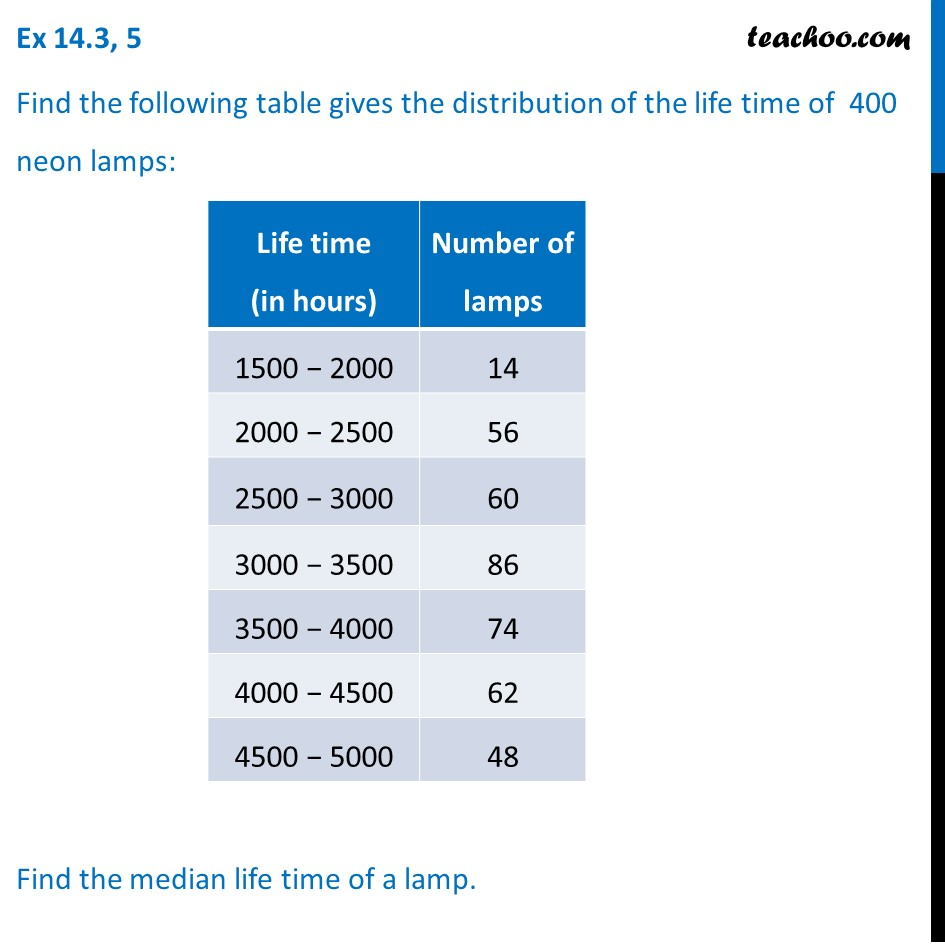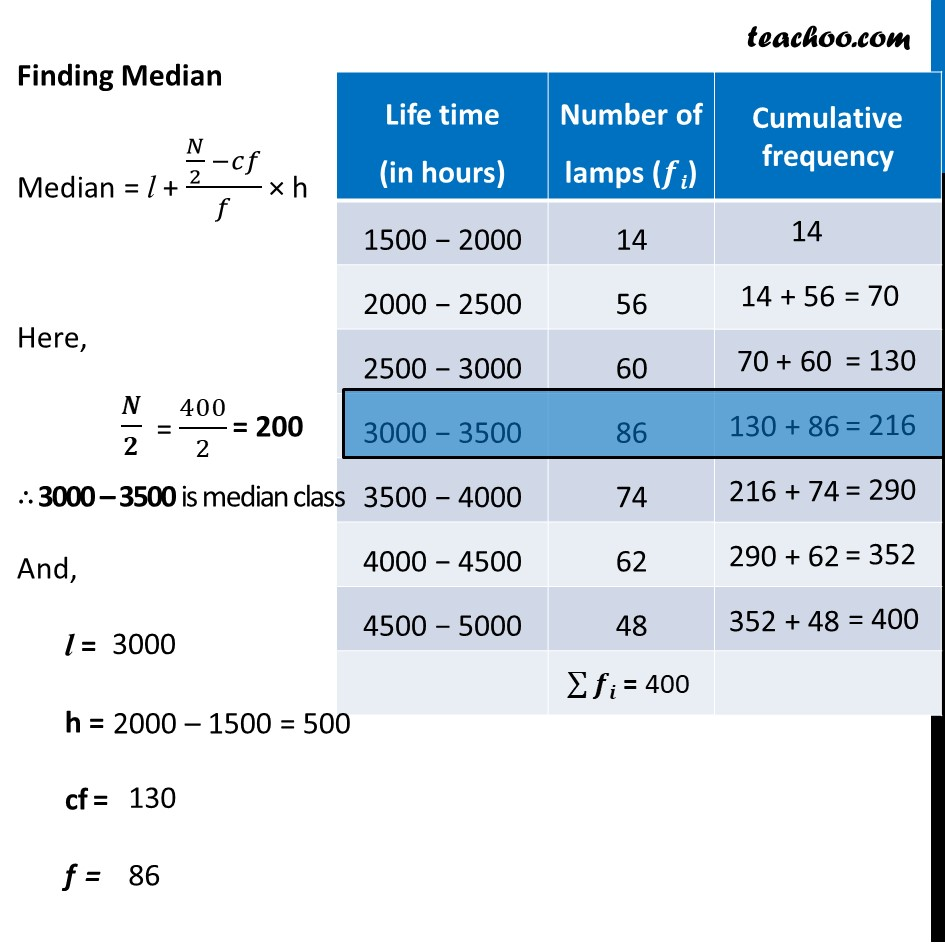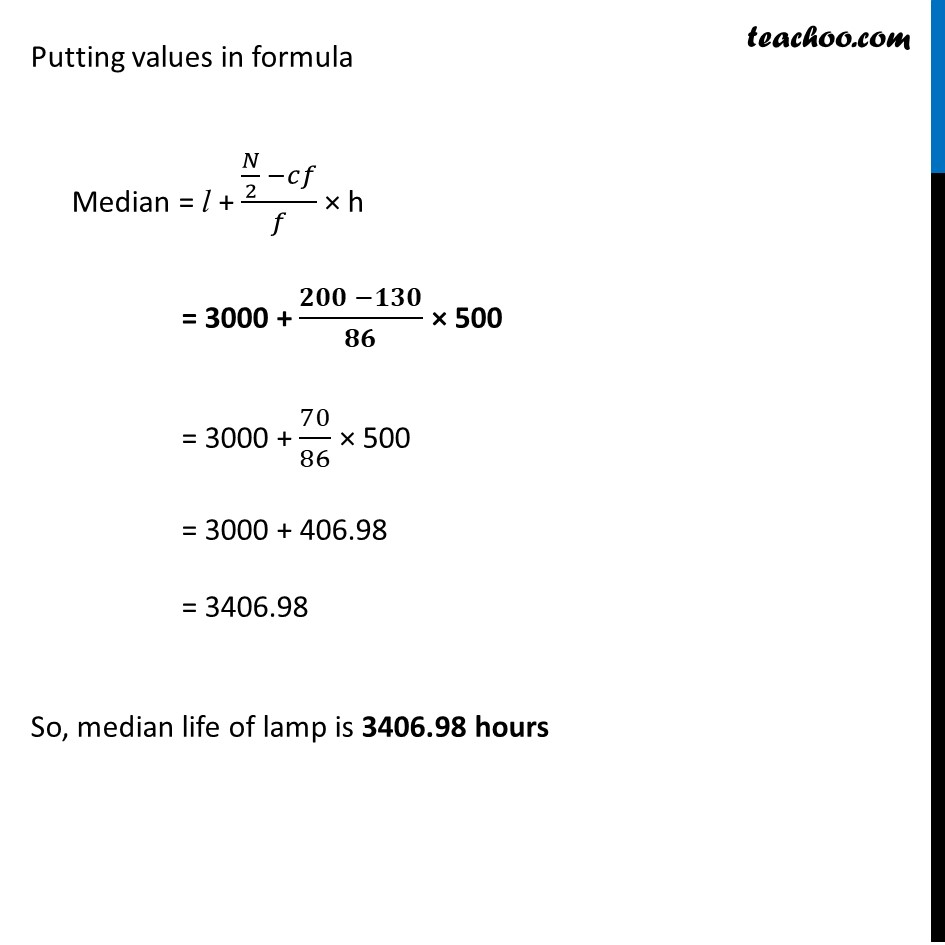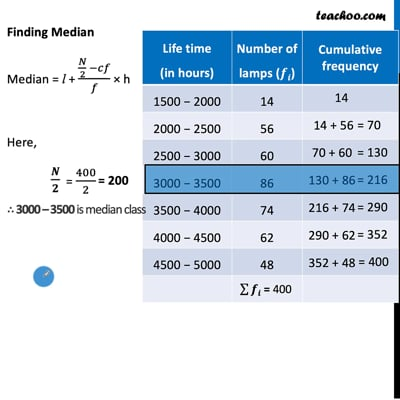Ex 14.3

Chapter 14 Class 10 Statistics
Serial order wiseThis video is only available for Teachoo black users

Solve all your doubts with Teachoo Black (new monthly pack available now!)

### Transcript

Ex 14.3, 5 Find the following table gives the distribution of the life time of 400 neon lamps: Find the median life time of a lamp. Finding Median Median = l + (𝑁/2 −𝑐𝑓)/𝑓 × h Here, 𝑵/𝟐 ∴ 3000 – 3500 is median class And, l = h = cf = f = 3000 2000 – 1500 = 500 Putting values in formula Median = l + (𝑁/2 −𝑐𝑓)/𝑓 × h = 3000 + (𝟐𝟎𝟎 −𝟏𝟑𝟎)/𝟖𝟔 × 500 = 3000 + 70/86 × 500 = 3000 + 406.98 = 3406.98 So, median life of lamp is 3406.98 hours